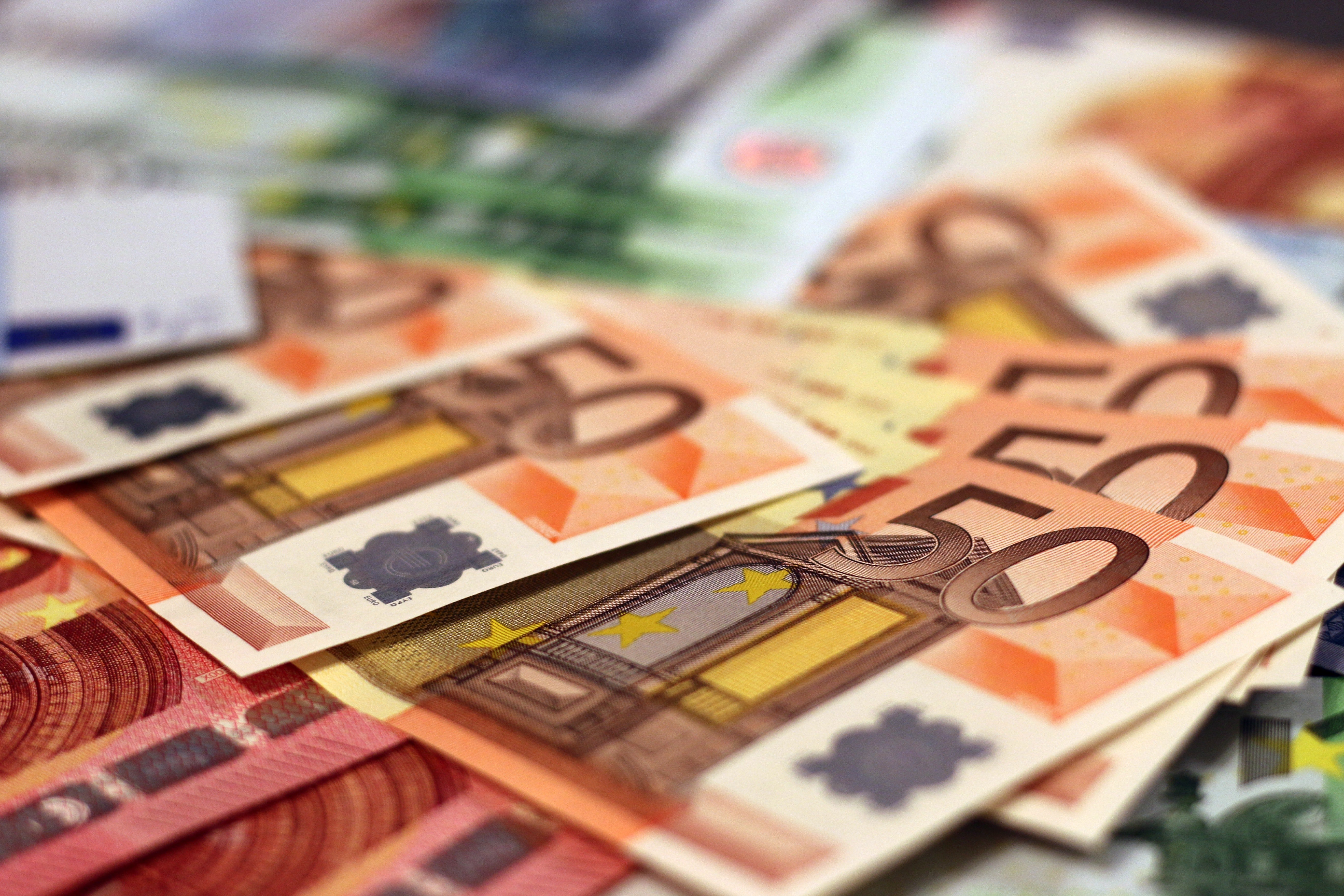﻿ Return On Investment (ROI) - Friedberg Direct# Return On Investment (ROI)

## What is ROI?

Return on investment (ROI) is a key concept for management of money that can be applied to business objectives as well as personal finance. In a basic sense, it's a calculation that tells an investor how well an investment is performing over time. However, the concept, in addition to the results the calculation of ROI produces, can vary somewhat depending on the variables taken into account and what time horizon is considered in making the calculation.

## How To Calculate ROI: The Basic Formula

The basic formula for calculating ROI is: ROI is equal to net profit or loss, divided by cost of investment. Mathematically, that is often expressed as:

ROI = (Gains from Investment - Cost of Investment) ÷ Cost of Investment

The definition of ROI generally looks and seems straightforward, and can be. However, the calculation of ROI will produce different results depending on how the terms of the equation, "cost of investment" or "gains from investment," are defined.

ROI can be calculated as a final value or a projected value, the latter also known as "expected return on investment." A projected return can be an educated guess of return, based on known and estimated future information. The final value will be based on actual returns once they have been verified.

## Some Variations On The Theme Of ROI

There are several formal methodologies used by businesses for calculating ROI, which include internal rate of return, net present value, payback and profitability indices. For practical purposes, however, a few basic conceptions that ROI follows:

1) Total Return

The total return expresses how much an investment has produced over a given period of time in a nominal money amount. This will take into account all forms of income from the investment, including any capital gains or losses, and any dividend or interest income.

2) Percentage Return

The percentage return can help investors understand how well an investment did in relation to the original amount they invested. This will equal the total return divided by the investment amount, multiplied by 100.

3) Average Annual Return

The average annual return uses the percentage return, but it also considers how long the investment is held. The calculation for the annual average return is:

Annual Return = (Simple Return Percentage +1) (1 / Years Held)-1

## Cash Flow Method

To account for possible changes over time, business investors often prefer use of cash flow methods that show return over a series of future periods, considering any possible changes in returns from year to year.

## Accounting For Costs

As with businesses, both individual and institutional investors will face costs in doing the business of managing their portfolios. These will include items like trading fees, taxes and interest charges.

To simplify this process, traders can subtract the costs from the final (i.e. "current") value of their investment when it is sold, and can conceive of the ROI calculation in this manner:

ROI = Current Value of Investment – Initial Value of Investment ÷ Initial Value of Investment

## ROI Errors

ROI figures, particularly within expected ROI, may be subject to errors. These may have to do with miscalculation of future profits or a neglect to include certain risks or costs associated with an investment.

## Currency Trading Profits And Costs

ROI in currency trading will take in all of the basic factors mentioned above. Naturally, gains from investment, or "current value," will depend on market conditions and the individual trader's ability. The costs in currency trading will include standard investment costs such as capital gains and income taxes. With trading itself, traders will be subject to broker commissions and spreads.

In some cases, commissions and spreads will be rolled into a single cost that is inserted into the spread (the difference between the bid and ask prices). In other cases, brokers may separate commission and spread costs in order to give more transparency to total trading costs for their clients.

## Summary

ROI is an important concept for investors whether they are making business investment decisions or managing financial portfolios. Ultimately, it can reveal whether a particular investment appears worthwhile or not.

The basic calculation is simple. However, it can be modified according to varied interpretations of its component cost and gains from investment, in addition to according to time horizons used for its calculation. Once investors decide the relevant values of these components for each investment, they will certainly benefit from using the concept to evaluate results and potential for investments in any and all asset categories.

### References

 Retrieved 07 Oct 2016 https://books.google.com/books Retrieved 07 Oct 2016 https://finance.zacks.com/types-return-investment-8135.html Retrieved 07 Oct 2016 https://www.businessinsider.com/how-to-calculate-a-return-on-investment-2010-1 Retrieved 07 Oct 2016 https://books.google.com/books